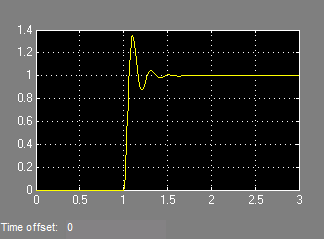# Importing variable-step signals into Simulink

I have a data acquisition system which saves signals at variable intervals. I want to import this data into a variable-step simulation. When I use the From Workspace block, the Simulink variable-step solver seems to take large steps and skip some of my points. How can I force the solver to take steps at the times specified in my input data?

Generating a variable-step signal

To illustrate this problem, I will generate data using one variable-step simulation and try to input it into another. To begin I simulate the following model using the ode15s stiff solver: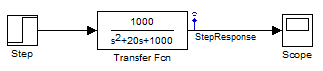When inspecting the logged data, we can see that the step size is not constant: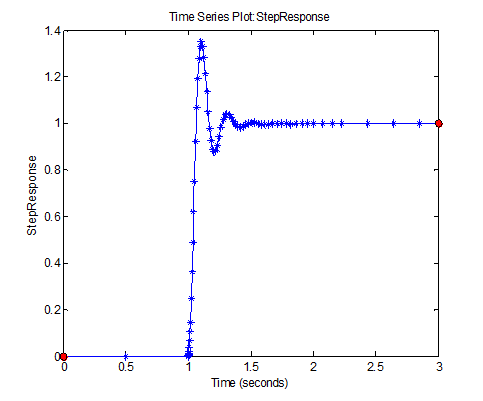Importing the signal into a model

To import this data in a simulation, I use a root-level Inport block.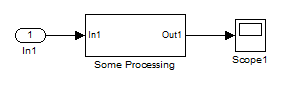Since the data to import is continuous, I enable the Interpolate option of the Inport block: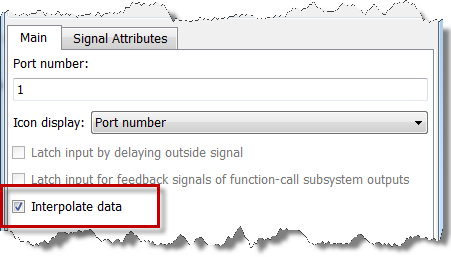Then I configure the model to use the saved data as input: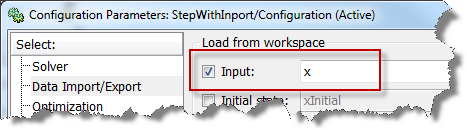Reproducing the problem

The Simulink variable-step solvers adjusts the step size during the simulation to achieve the specified tolerance for state error as defined in the model configuration. The variable-step solver does not have to take steps at the times specified in the imported data. In my example model, when I run the simulation, the imported signal looks like: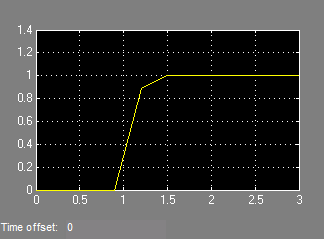The solution

We need to instruct the Simulink variable-step solver to execute at the times specified by the imported data. To do this, open the Data Import/Export configuration pane and look at the Save options section. Set the Output options to “Produce additional output”, and specify the time points of the imported data as the Output times.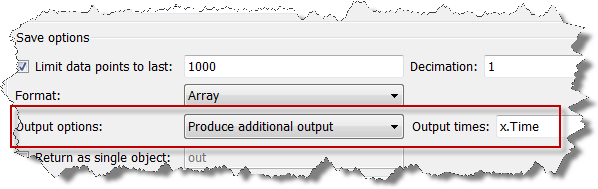Now the imported data looks as expected.